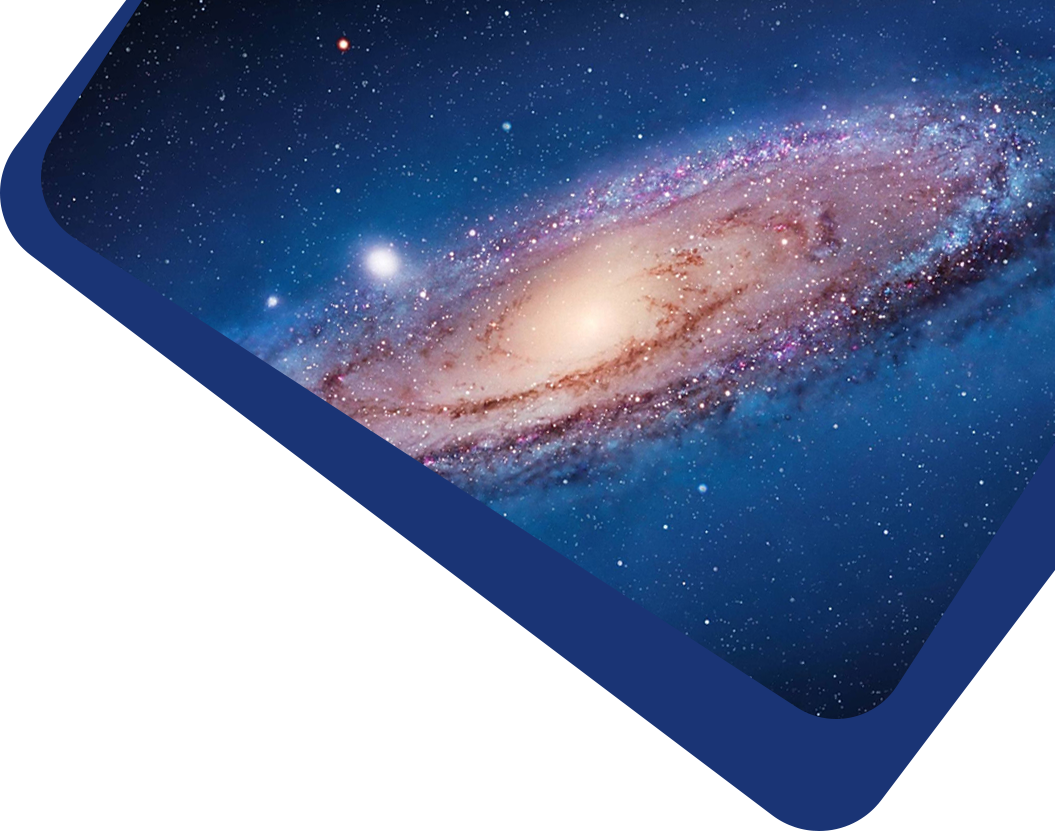Vol 23, No 8

## Velocity Dispersion σ_{aper} Aperture Corrections as a Function of Galaxy Properties from Integral-field Stellar Kinematics of 10,000 MaNGA Galaxies

#### Kai Zhu, Ran Li, Xiaoyue Cao, Shengdong Lu, Michele Cappellari and Shude Mao

Abstract

The second moment of the stellar velocity within the effective radius, denoted by $$\sigma_{\rm e}^2$$, is a crucial quantity in galaxy studies, as it provides insight into galaxy properties and their mass distributions. However, large spectroscopic surveys typically do not measure σe directly, instead providing σaper, the second moment of the stellar velocity within a fixed fiber aperture. In this paper, we derive an empirical aperture correction formula, given by $$\sigma_{\rm aper}/\sigma_{\rm e}=(R_{\rm aper}/R_{\rm e})^{\alpha}$$, using spatially resolved stellar kinematics extracted from approximately 10,000 Sloan Digital Sky Survey–Mapping Nearby Galaxies at Apache Point Observatory integral field unit observations. Our analysis reveals a strong dependence of α on the r-band absolute magnitude Mr, gi color, and Sérsic index nSer, where α values are lower for brighter, redder galaxies with higher Sérsic indices. Our results demonstrate that the aperture correction derived from previous literature on early-type galaxies cannot be applied to predict the aperture corrections for galaxies with intermediate Sérsic indices. We provide a lookup table of α values for different galaxy types, with parameters in the ranges of −18 > Mr > −24, 0.4 < gi < 1.6, and 0 < nSer < 8. A Python script is provided to obtain the correction factors from the lookup table.

Keywords

Full Text
Refbacks